## Triangles¶

### Right triangle¶

In a right triangle, one angle is the same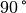, the other two angles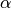and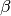result together.

The following applies to the area and the circumference of a right triangle: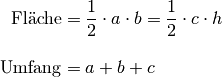The Pythagorean theorem

Right-angled triangles have a special feature: if you square the lengths of the sides of the triangle, the square number corresponds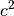the longest side of the triangle (the "hypotenuse") exactly the sum of the square numbers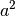and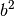the shorter sides of the triangle (the "cathetus").

This law, known as the “Pythagorean theorem”, can be graphically illustrated by running along the hypotenuse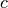and the two catheters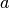and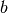Draws squares with the corresponding side lengths and compares the areas with each other: The areas of the two smaller squaresandare with the big squaresurface area.

In practice, the Pythagorean theorem proves to be useful to work around two boards, poles, etc. of known lengthsandto be arranged at right angles to each other. Solve equation (3) for the length of the connecting lineon, so it turns out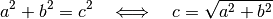Are the corner points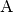and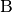exactly around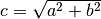apart, the angle is betweenandexactly. The length ratio is particularly suitable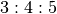because here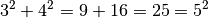applies; the length of the base unit can be freely selected.

Elevation and cathetus set

In addition, two other relationships apply in the right triangle:

• Height rate:

The product of the two parts of the hypotenuse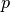and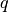the right and left of the height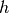is equal to the square of the height: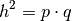• Cathetus set: The product of a cathetus is equal to the product of the hypotenuseand the adjacent hypotenuse: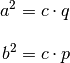These two laws were already discovered by Euclid. They are based on the triangles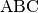and the two by the heightresulting triangles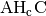and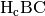are similar to each other: All contain a right angle and each have one side of the triangle in common; in addition, all triangles have the angle because of equation (1)together.

Due to the similarity, the proportions of the side lengths are the same, it applies, for example, to the triangles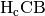andthe aspect ratio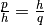, which also turns out to be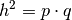can be written and thus corresponds to the height rate. The two sets of cathets also follow from the length ratios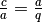of trianglesandas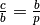of trianglesand.

Other properties

Further relationships in triangles are discussed in more detail in the Trigonometry section.

Remarks: